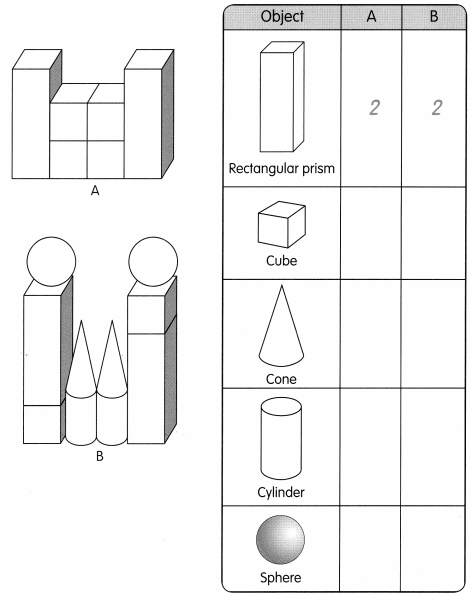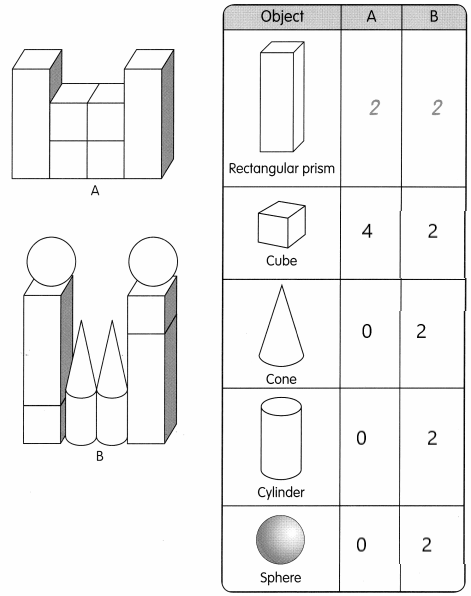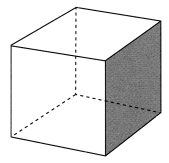# Math in Focus Grade 2 Chapter 19 Practice 2 Answer Key Solid Shapes

This handy Math in Focus Grade 2 Workbook Answer Key Chapter 19 Practice 2 Solid Shapes detailed solutions for the textbook questions.

## Math in Focus Grade 2 Chapter 19 Practice 2 Answer Key Solid Shapes

Write the number of solid shapes used in each model.

Question 1.Explanation:
I wrote the number of solid shapes used in each model
There are 2 rectangular prisms in both shapes A and B
There are 4 cubes in shape A and 2 cubes in shape B
There are 0 cones in shape A and 2 cones in shape B
There are 0 cylinder in shape A and 2 cylinders in shape B
There are 0 spheres in shape A and 2 spheres in shapes B.

Look at the cube.Question 2.
How many faces can you see on the cube?